# Math 9 similar triangles intro

7 de Mar de 2015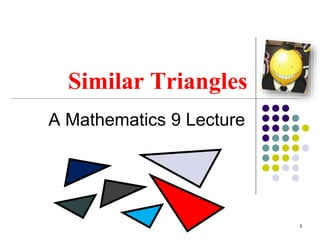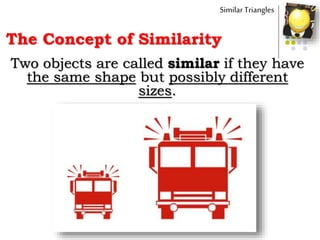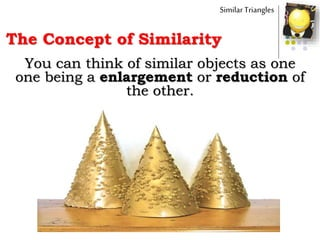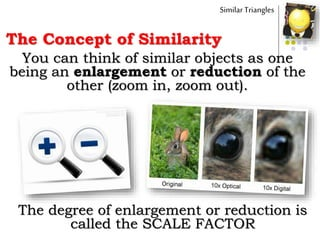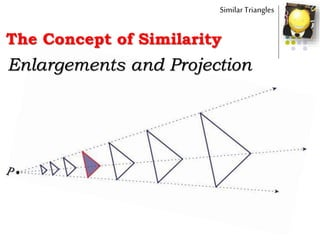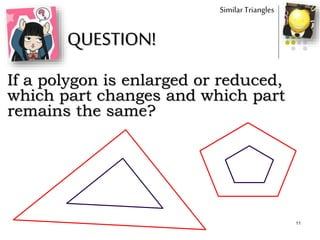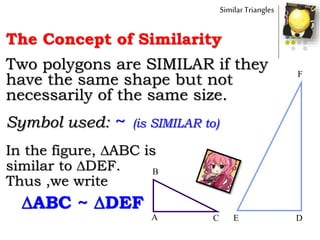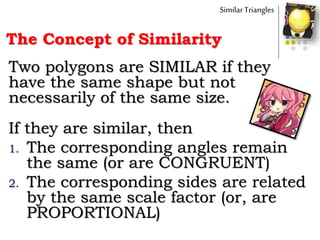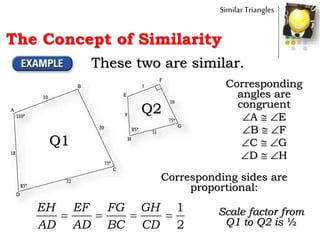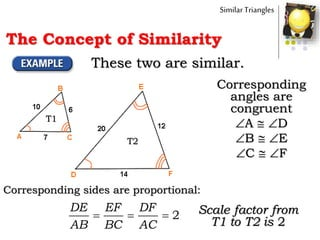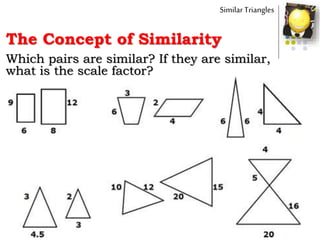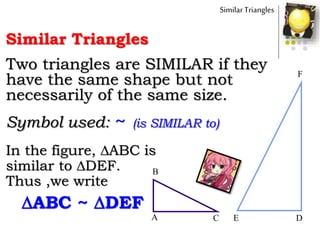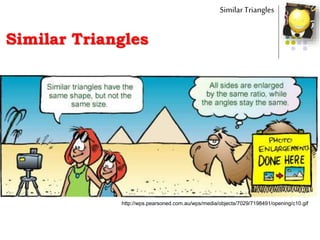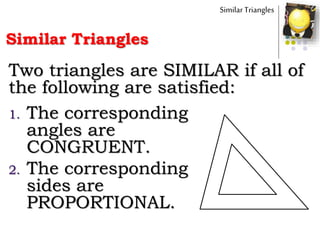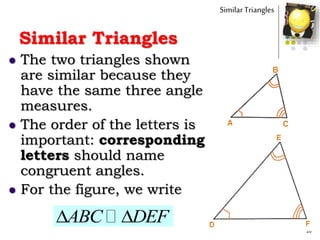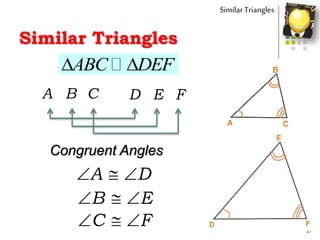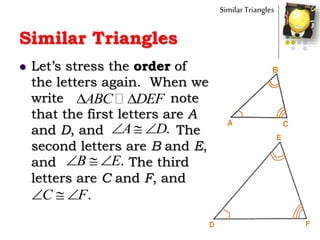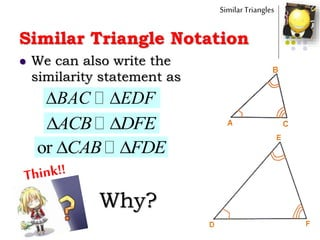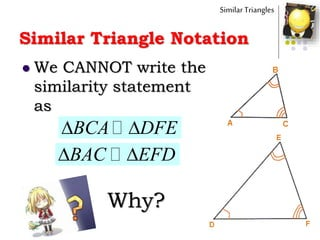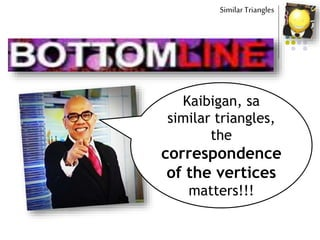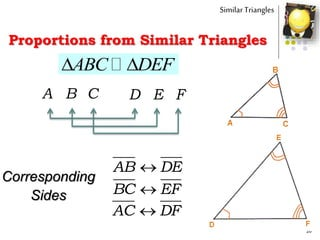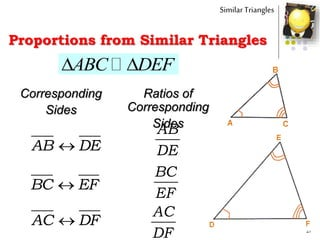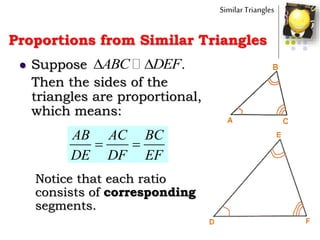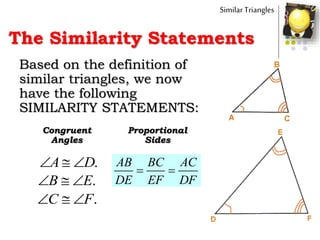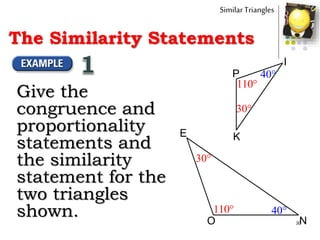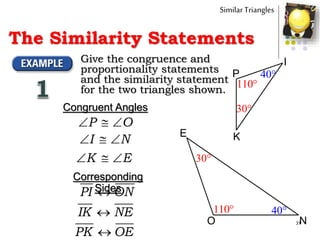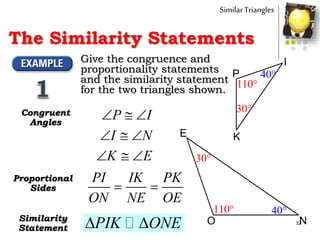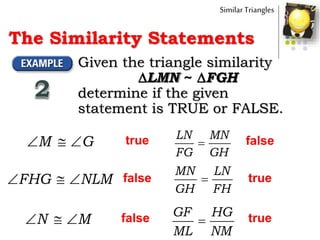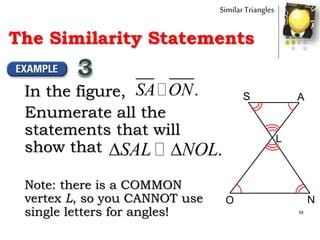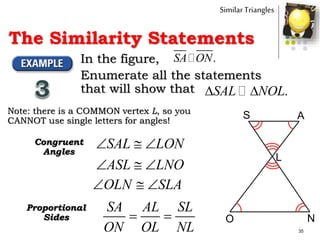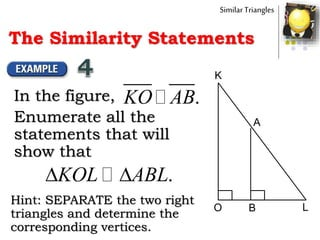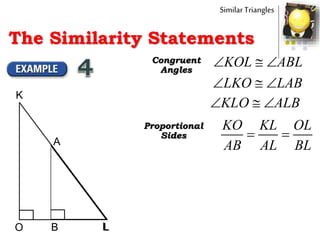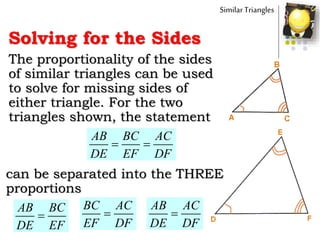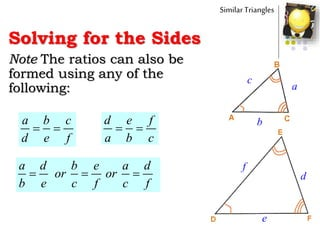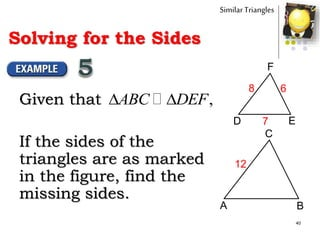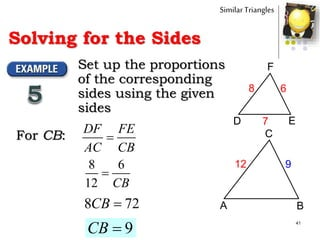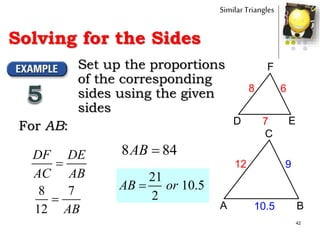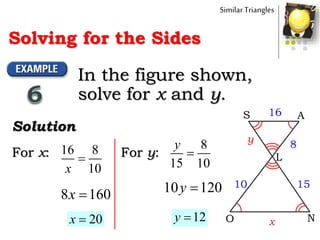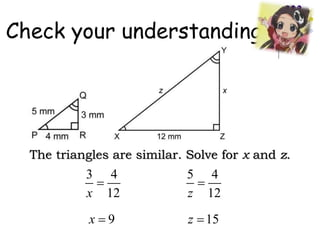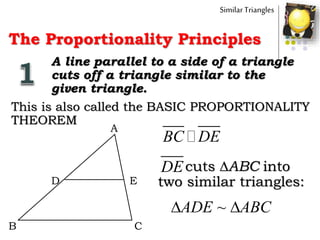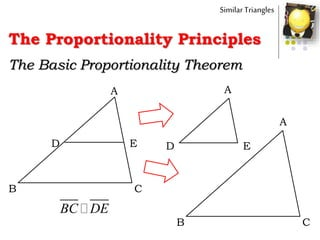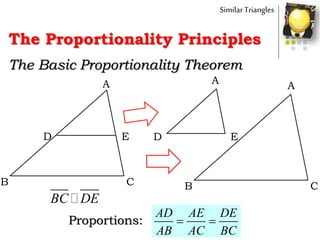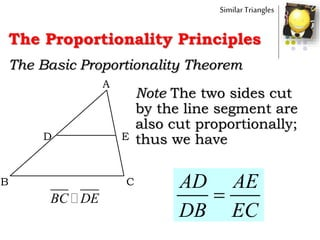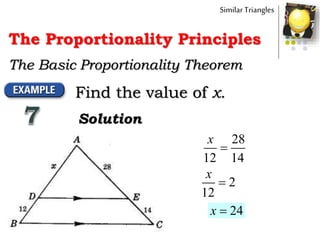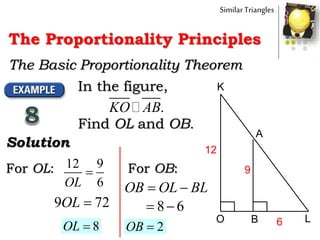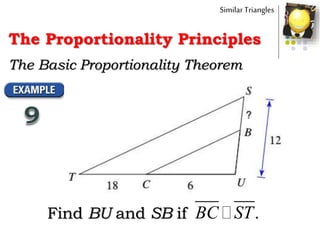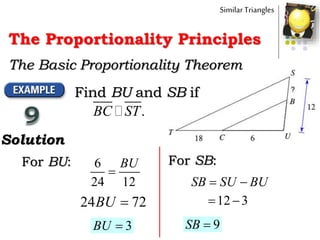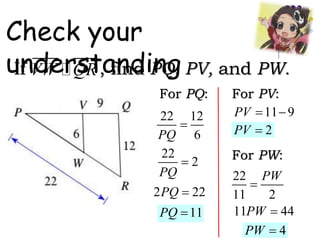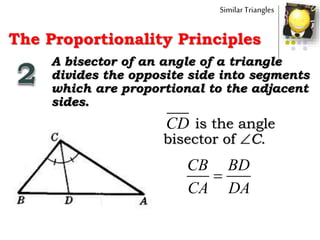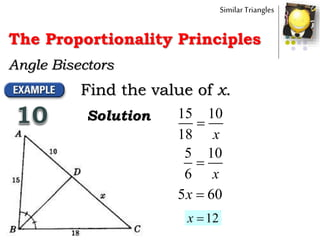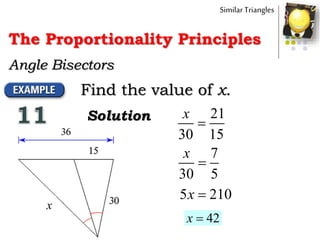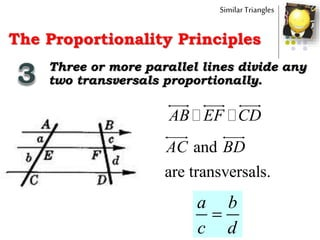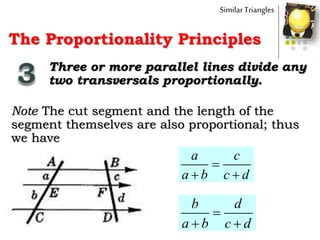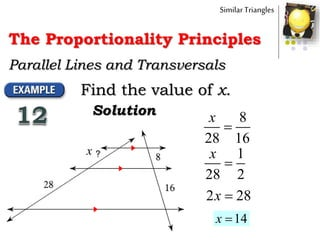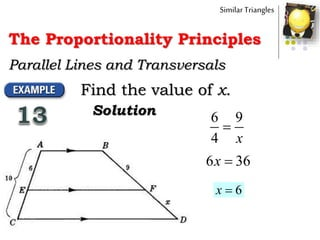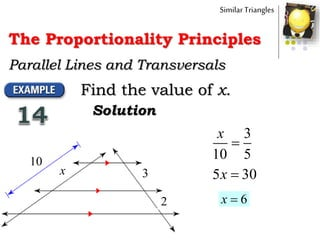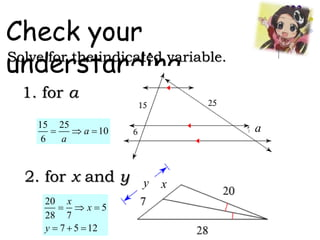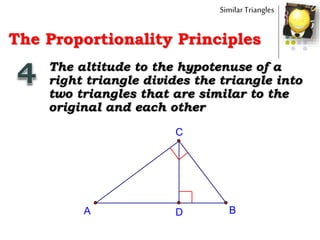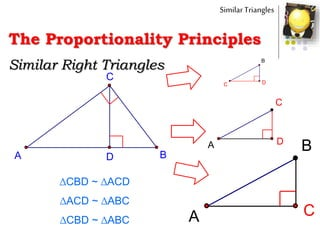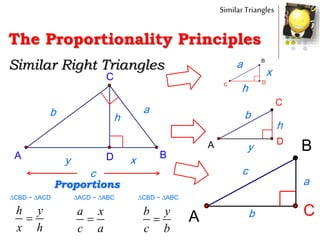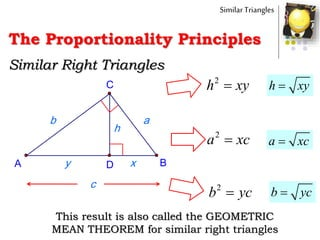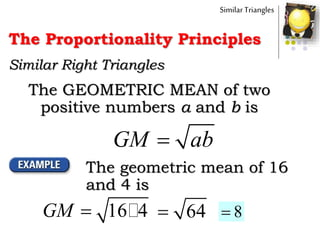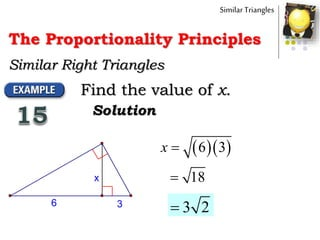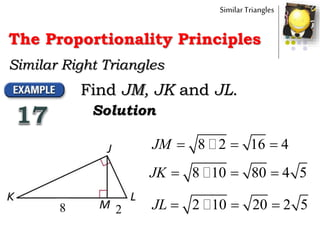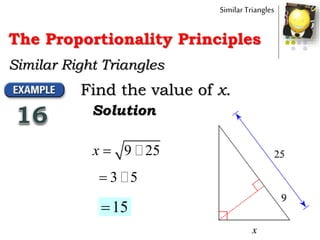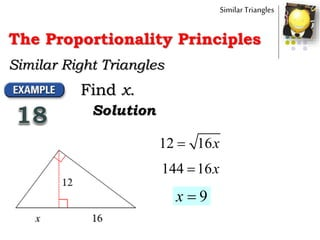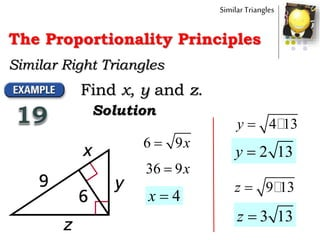1 de 74

### Math 9 similar triangles intro

• 1. The session shall begin shortly…
• 4. 4 Similar Triangles What do these pairs of objects have in common? SAME SHAPES BUT DIFFERENT SIZES
• 5. 5 Similar Triangles What do these pairs of objects have in common? They are also called SIMILAR objects
• 6. The Concept of Similarity Similar Triangles Two objects are called similar if they have the same shape but possibly different sizes.
• 7. The Concept of Similarity Similar Triangles You can think of similar objects as one one being a enlargement or reduction of the other.
• 8. The Concept of Similarity Similar Triangles You can think of similar objects as one being an enlargement or reduction of the other (zoom in, zoom out). The degree of enlargement or reduction is called the SCALE FACTOR
• 10. The Concept of Similarity Similar Triangles Enlargements and Projection
• 11. 11 Similar Triangles QUESTION! If a polygon is enlarged or reduced, which part changes and which part remains the same?
• 12. The Concept of Similarity Similar Triangles Two polygons are SIMILAR if they have the same shape but not necessarily of the same size. Symbol used: ~ (is SIMILAR to) A C B DE F In the figure, ABC is similar to DEF. Thus ,we write ABC ~ DEF
• 13. The Concept of Similarity Similar Triangles Two polygons are SIMILAR if they have the same shape but not necessarily of the same size. If they are similar, then 1. The corresponding angles remain the same (or are CONGRUENT) 2. The corresponding sides are related by the same scale factor (or, are PROPORTIONAL)
• 14. The Concept of Similarity Similar Triangles Q1 Q2 These two are similar. Corresponding angles are congruent A  E B  F C  G D  H Corresponding sides are proportional: 1 2 EH EF FG GH AD AD BC CD     Scale factor from Q1 to Q2 is ½
• 15. The Concept of Similarity Similar Triangles T1 T2 These two are similar. Corresponding angles are congruent A  D B  E C  F Corresponding sides are proportional: 2 DE EF DF AB BC AC    Scale factor from T1 to T2 is 2
• 16. Similar Triangles The Concept of Similarity Which pairs are similar? If they are similar, what is the scale factor?
• 17. Similar Triangles Similar Triangles Two triangles are SIMILAR if they have the same shape but not necessarily of the same size. Symbol used: ~ (is SIMILAR to) A C B DE F In the figure, ABC is similar to DEF. Thus ,we write ABC ~ DEF
• 19. Similar Triangles Two triangles are SIMILAR if all of the following are satisfied: 1. The corresponding angles are CONGRUENT. 2. The corresponding sides are PROPORTIONAL. Similar Triangles
• 20. Similar Triangles  The two triangles shown are similar because they have the same three angle measures.  The order of the letters is important: corresponding letters should name congruent angles.  For the figure, we write 20 ABC DEF  Similar Triangles
• 21. Similar Triangles 21 ABC DEF  Similar Triangles A B C D E F Congruent Angles A D   B E   C F  
• 22.  Let’s stress the order of the letters again. When we write note that the first letters are A and D, and The second letters are B and E, and The third letters are C and F, and 22 ABC DEF  .A D   .B E   .C F   Similar Triangles Similar Triangles
• 23.  We can also write the similarity statement as 23 ACB DFE   BAC EDF or CAB FDE  Similar Triangle Notation Similar Triangles Why?
• 24.  BCA DFE Similar Triangle Notation Similar Triangles  We CANNOT write the similarity statement as  BAC EFD Why?
• 25. Kaibigan, sa similar triangles, the correspondence of the vertices matters!!! Similar Triangles
• 26. 26 ABC DEF  Similar Triangles A B C D E F Corresponding Sides AB DE BC EF AC DF Proportions from Similar Triangles
• 27. 27 ABC DEF  Similar Triangles Corresponding Sides AB DE BC EF AC DF Proportions from Similar Triangles Ratios of Corresponding Sides AB DE BC EF AC DF
• 28.  Suppose Then the sides of the triangles are proportional, which means: 28 .ABC DEF  AB AC BC DE DF EF   Notice that each ratio consists of corresponding segments. Similar Triangles Proportions from Similar Triangles
• 29. The Similarity Statements Based on the definition of similar triangles, we now have the following SIMILARITY STATEMENTS: 29 Congruent Angles .A D   .B E   .C F   Proportional Sides Similar Triangles   AB BC AC DE EF DF
• 30. 30O N E P K I 110 110 30 30 40 40 Similar Triangles Give the congruence and proportionality statements and the similarity statement for the two triangles shown. The Similarity Statements
• 31. The Similarity Statements 31O N E P K I 110 110 30 30 40 40 Similar Triangles Give the congruence and proportionality statements and the similarity statement for the two triangles shown. Congruent Angles P O   I N   K E   Corresponding SidesPI ON IK NE PK OE
• 32. 32O N E P K I 110 110 30 30 40 40 Similar Triangles Give the congruence and proportionality statements and the similarity statement for the two triangles shown. Congruent Angles   P I   I N   K E Proportional Sides   PI IK PK ON NE OE Similarity Statement  PIK ONE The Similarity Statements
• 33. Similar Triangles Given the triangle similarity LMN ~ FGH determine if the given statement is TRUE or FALSE. M G   true FHG NLM   false N M   false LN MN FG GH  false MN LN GH FH  true GF HG ML NM  true The Similarity Statements
• 34. In the figure, Enumerate all the statements that will show that 34 .SA ON S A L O N . SAL NOL Similar Triangles The Similarity Statements Note: there is a COMMON vertex L, so you CANNOT use single letters for angles!
• 35. In the figure, Enumerate all the statements that will show that 35 .SA ON S A L O N . SAL NOL Similar Triangles The Similarity Statements Congruent Angles   SAL LON   ASL LNO   OLN SLA Proportional Sides   SA AL SL ON OL NL Note: there is a COMMON vertex L, so you CANNOT use single letters for angles!
• 36. Similar Triangles In the figure, Enumerate all the statements that will show that .KO AB . KOL ABL O B L K A Hint: SEPARATE the two right triangles and determine the corresponding vertices. Similar Triangles The Similarity Statements
• 37. O B L K A Similar TrianglesSimilar Triangles The Similarity Statements O L K Congruent Angles   KOL ABL   LKO LAB   KLO ALB Proportional Sides   KO KL OL AB AL BL
• 38. Similar Triangles Solving for the Sides The proportionality of the sides of similar triangles can be used to solve for missing sides of either triangle. For the two triangles shown, the statement 38   AB BC AC DE EF DF can be separated into the THREE proportions  AB AC DE DF  BC AC EF DF  AB BC DE EF
• 39. Similar Triangles Solving for the Sides Note The ratios can also be formed using any of the following: 39 a b c d e f   a b c d e f   d e f a b c    a d b e a d or or b e c f c f
• 40. Given that If the sides of the triangles are as marked in the figure, find the missing sides. 40 A B C D E F ,ABC DEF  68 7 12 Similar Triangles Solving for the Sides
• 41. 41 A B C D E F 68 7 12 9  DF FE AC CB Similar Triangles Solving for the Sides Set up the proportions of the corresponding sides using the given sides For CB: 8 6 12  CB 8 72CB 9CB
• 42. 42 A B C D E F 68 7 12 9 10.5 Similar Triangles Solving for the Sides Set up the proportions of the corresponding sides using the given sides  DF DE AC AB For AB: 8 7 12  AB 8 84AB 21 10.5 2 AB or
• 43. S A L O N 8 10 16 x y Similar Triangles Solving for the Sides In the figure shown, solve for x and y. Solution 15 16 8 10  x For x: 8 160x 20x 8 15 10  y For y: 10 120y 12y
• 44. Check your understanding The triangles are similar. Solve for x and z. 3 4 12  x 9x 5 4 12  z 15z
• 45. Similar Triangles The Proportionality Principles A line parallel to a side of a triangle cuts off a triangle similar to the given triangle. This is also called the BASIC PROPORTIONALITY THEOREM BC DE cuts ABC into two similar triangles: DE ~ ADE ABC A B C D E
• 46. A B C D E Similar Triangles The Proportionality Principles The Basic Proportionality Theorem A D E B C A BC DE
• 47. A B C D E Similar Triangles The Proportionality Principles The Basic Proportionality Theorem A D E B C A BC DE   AD AE DE AB AC BC Proportions:
• 48. A B C D E Similar Triangles The Proportionality Principles The Basic Proportionality Theorem BC DE  AD AE DB EC Note The two sides cut by the line segment are also cut proportionally; thus we have
• 49. Similar Triangles The Proportionality Principles The Basic Proportionality Theorem Find the value of x. Solution 28 12 14  x 2 12  x 24x
• 50. Similar Triangles The Proportionality Principles The Basic Proportionality Theorem O B L K A 12 6 9 In the figure, Find OL and OB. .KO AB Solution 12 9 6  OL For OL: 9 72OL 8OL For OB:  OB OL BL 8 6  2OB
• 51. Similar Triangles The Proportionality Principles The Basic Proportionality Theorem Find BU and SB if .BC ST
• 52. Similar Triangles The Proportionality Principles The Basic Proportionality Theorem Find BU and SB if .BC ST Solution 6 24 12  BUFor BU: 24 72BU 3BU  SB SU BU For SB: 12 3  9SB
• 53. Check your understandingIf , find PQ, PV, and PW.VW QR 22 12 6  PQ For PQ: 22 2 PQ 2 22PQ 11PQ For PV: 11 9 PV 2PV 22 11 2  PW For PW: 11 44PW 4PW
• 54. Similar Triangles The Proportionality Principles A bisector of an angle of a triangle divides the opposite side into segments which are proportional to the adjacent sides. is the angle bisector of C. CD  CB BD CA DA
• 55. Similar Triangles The Proportionality Principles Angle Bisectors Find the value of x. Solution 15 10 18  x 5 10 6  x 5 60x 12x
• 56. Similar Triangles The Proportionality Principles Angle Bisectors Find the value of x. Solution 21 30 15  x 7 30 5  x 5 210x 42x x
• 57. Similar Triangles The Proportionality Principles Three or more parallel lines divide any two transversals proportionally. AB EF CD and are transversals. AC BD  a b c d
• 58. Similar Triangles The Proportionality Principles Three or more parallel lines divide any two transversals proportionally. Note The cut segment and the length of the segment themselves are also proportional; thus we have    a c a b c d    b d a b c d
• 59. Similar Triangles The Proportionality Principles Parallel Lines and Transversals Find the value of x. Solution 8 28 16  x 1 28 2  x 2 28x 14x x
• 60. Similar Triangles The Proportionality Principles Parallel Lines and Transversals Find the value of x. Solution 6 9 4  x 6 36x 6x
• 61. Similar Triangles The Proportionality Principles Parallel Lines and Transversals Find the value of x. Solution 3 10 5  x x 10 3 2 5 30x 6x
• 62. Check your understandingSolve for the indicated variable. 2. for x and y y 20 5 28 7 7 5 12       x x y 1. for a a15 25 10 6   a a x
• 63. Similar Triangles The Proportionality Principles The altitude to the hypotenuse of a right triangle divides the triangle into two triangles that are similar to the original and each other A B C D
• 64. Similar Triangles The Proportionality Principles A B C D C D B A D C A C B ∆CBD ~ ∆ACD ∆ACD ~ ∆ABC ∆CBD ~ ∆ABC Similar Right Triangles
• 65. Similar Triangles The Proportionality Principles A B C D C D B A D C A C B Similar Right Triangles h ab y x c x h h y a b a b c  h y x h  a x c a  b y c b Proportions ∆CBD ~ ∆ACD ∆ACD ~ ∆ABC ∆CBD ~ ∆ABC
• 66. Similar Triangles The Proportionality Principles A B C D h ab y x c 2 h xy h xy 2 a xc a xc 2 b yc b yc Similar Right Triangles This result is also called the GEOMETRIC MEAN THEOREM for similar right triangles
• 67. Similar Triangles The Proportionality Principles Similar Right Triangles The GEOMETRIC MEAN of two positive numbers a and b is GM ab The geometric mean of 16 and 4 is 16 4GM 64 8
• 68. Similar Triangles The Proportionality Principles Similar Right Triangles Find the value of x. Solution 36 x   6 3x 18 3 2
• 69. Similar Triangles The Proportionality Principles Similar Right Triangles Find JM, JK and JL. Solution 8 2 8 2 16 4  JM 8 10 80 4 5  JK 2 10 20 2 5  JL
• 70. Similar Triangles The Proportionality Principles Similar Right Triangles Find the value of x. Solution 9 25x 3 5 15
• 71. Similar Triangles The Proportionality Principles Similar Right Triangles Find x. Solution 12 16 x 144 16 x 9x
• 72. Similar Triangles The Proportionality Principles Similar Right Triangles Find x, y and z. Solution 6 9 x 36 9 x 4x 4 13y 2 13y 9 13z 3 13z